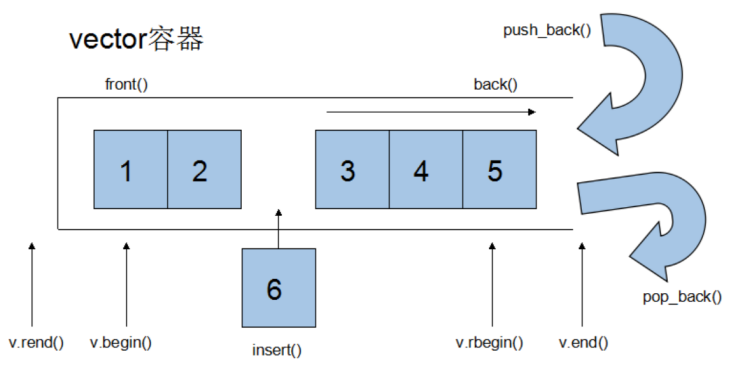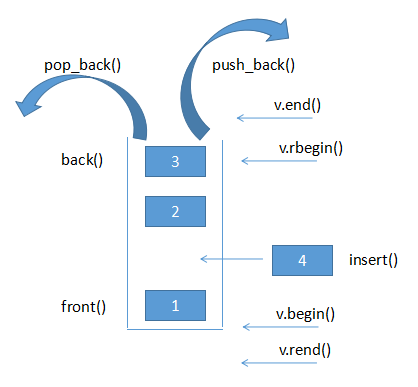• vector容器 1.vector基本概念 vector容器与数组array非常的相似，两者的区别主要在于空间使用的灵活性，Array是静态空间，一旦配置就不能更改。vector是动态空间，随着元素的增加，它的内部机制会自动的扩充空间以...
vector容器
1.vector基本概念
vector容器与数组array非常的相似，两者的区别主要在于空间使用的灵活性，Array是静态空间，一旦配置就不能更改。vector是动态空间，随着元素的增加，它的内部机制会自动的扩充空间以容纳新的元素。因此vector的运用可以对内存有合理和灵活的运用。2.vector数据结构
Vector所采用的数据结构非常简单，线性连续空间，它以两个迭代器_Myfirst和_Mylast分别指向配置得来的连续空间中目前已被使用的范围，并以迭代器_Myend指向整块连续内存空间的尾端。
注意：一个vector的容量永远大于或等于其大小，一旦容量等于大小，便是满载，下次再有新增元素，整个vector容器就得另觅居所。
对vector的任何操作，一旦引起空间的重新配置，指向原vector的所有迭代器就都失效了。
** 3. vector常用API操作**
#define _CRT_SECURE_N0_WARNINGS
#include<iostream>
#include <vector>
using namespace std;

void test01()
{

vector<int> v;
for(int i=0;i<10;i++)
{
v.push_back(i);
cout<<v.capacity()<<endl; //v.capacity()容器的容量
}
}
/*
//vector大小操作
size（）返回容器中元素的个数
empty（）判断容器是否为空
capacity（）容器的容量
reserve（int len）容器预留len个元素的长度预留位置不初始化元素不可访问

*/
void printVector(vector <int>&v)
{
for(vector<int>::iterator it=v.begin();it!=v.end();it++)
{
cout<<*it<<"";

}
cout<<endl;

}
void test02()
{
vector<int >v;
int arr[]={1,2,3,4,5,6};
vector<int>v1(arr,arr+sizeof(arr)/sizeof(int));

vector<int>v2(v1.begin(),v1.end());
printVector(v2);
vector<int> v3(10,100);
printVector(v3);

//赋值的使用
//assign(beg, end);//将[beg, end)区间中的数据拷贝赋值给本身。
vector<int>v4;
v4.assign(v3.begin(),v3.end());
printVector(v4);

//巧用swap  swap(vec);// 将vec与本身的元素互换。
v4.swap(v2);
cout<<"交换后的V4:"<<endl;
printVector(v4);
}
void test03()
{
vector<int>v;
for(int i=0;i<1000;i++)
{

v.push_back(i);
}
cout<<"v的容量:"<<v.capacity()<<endl;
cout<<"v的大小:"<<v.size()<<endl;
v.resize(3);
cout<<"v的容量:"<<v.capacity()<<endl;
cout<<"v的大小:"<<v.size()<<endl;

}
int main()
{
// test01();
test02();
//	test03();
system("pause");
return EXIT_SUCCESS;
}

1）vector大小操作
size();//返回容器中元素的个数
empty();//判断容器是否为空
resize(int num);//重新指定容器的长度为num，若容器变长，则以默认值填充新位置。如果容器变短，则末尾超出容器长度的元素被删除。
resize(int num, elem);//重新指定容器的长度为num，若容器变长，则以elem值填充新位置。如果容器变短，则末尾超出容器长>度的元素被删除。
capacity();//容器的容量
reserve(int len);//容器预留len个元素长度，预留位置不初始化，元素不可访问。

2） vector数据存取操作
at(int idx); //返回索引idx所指的数据，如果idx越界，抛出out_of_range异常。
operator[];//返回索引idx所指的数据，越界时，运行直接报错
front();//返回容器中第一个数据元素
back();//返回容器中最后一个数据元素代码片



vector插入和删除操作

insert(const_iterator pos, int count,ele);//迭代器指向位置pos插入count个元素ele.
push_back(ele); //尾部插入元素ele
pop_back();//删除最后一个元素
erase(const_iterator start, const_iterator end);//删除迭代器从start到end之间的元素
erase(const_iterator pos);//删除迭代器指向的元素
clear();//删除容器中所有元素



展开全文• 1.vector容器示意图 2.vector的迭代器 vector支持随机存取，所以vector提供的是随机访问迭代器。 3.vector的数据结构 vector采用连续线性空间实现，它用两个迭代器_Myfirst和_Mylast分别指向配置得来的连续...
1.vector容器示意图2.vector的迭代器
vector支持随机存取，所以vector提供的是随机访问迭代器。
3.vector的数据结构
vector采用连续线性空间实现，它用两个迭代器_Myfirst和_Mylast分别指向配置得来的连续空间中目前已被使用的范围，并以迭代器_Myend指向整块连续内存空间的尾端。一个vector的容量永远大于或等于其大小，一旦容量等于大小，便是满载，下次再有新增元素，整个vector容器就另外开辟内存。
4.vector常用API
4.1vector构造函数
vector<T> v;采用模板类实现，默认构造函数
vector（v.begin(),v.end()）；将[v.begin(),v.end())区间中的元素拷贝给本身
vector（n,elem）；构造函数将n个elem拷贝给本身。
vector（const vector& v）;拷贝构造
4.2vector赋值操作
assign（v.begin(),b.end()）;将[begin,end)区间中的数据拷贝赋值给本身
assign（n,elem）;将nge elem拷贝赋值给本身
vector& operator=（const vector& vec）;重载=
swap(vec);将vect与本身的元素互换
4.3vector大小操作
size();返回元素个数
empty();判断是否为空
resize(int num);重置容器大小，若容器变短，超出容器长度的元素被删除，若容器变长，则以默认值填充
resize(int num,elem)；重置容器大小，若容器变短，超出容器长度的元素被删除，若容器变长，则以elem填充新位置
capacity（）；返回容器容量
reserve（int len）；容器预留len个元素长度，预留位置不初始化。
4.4vector数据存取
at(int idx);返回索引idx所指的数据，idx越界，跑出out_of_range异常
operator[]；[]重载
front();返回容器中第一个数据元素
back();返回容器中最后一个数据元素
4.5vector插入和删除
insert（const_iterator pos,int count,ele）;迭代器指向位置pos插入count个元素ele
push_back(ele);尾插
pop_back();删除最后一个元素
erase(const_iterator start,const_itrator end);删除迭代器从start到end之间的元素
erase（const_iterator pos）;删除迭代器指向的元素
clear();删除容器中所有的元素

展开全文• vector容器类型 vector容器是一个模板类，可以存放任何类型的对象（但必须是同一类对象）。vector对象可以在运行时高效地添加元素，并且vector中元素是连续存储的。 vector的构造 函数原型： template explicit ...
vector容器类型
vector容器是一个模板类，可以存放任何类型的对象（但必须是同一类对象）。vector对象可以在运行时高效地添加元素，并且vector中元素是连续存储的。
vector的构造

函数原型：
template<typename T>
explicit vector();                                 // 默认构造函数，vector对象为空
explicit vector(size_type n, const T& v = T());    // 创建有n个元素的vector对象
vector(const vector& x);
vector(const_iterator first, const_iterator last);

注：vector容器内存放的所有对象都是经过初始化的。如果没有指定存储对象的初始值，那么对于内置类型将用0初始化，对于类类型将调用其默认构造函数进行初始化（如果有其它构造函数而没有默认构造函数，那么此时必须提供元素初始值才能放入容器中）。

举例：
vector<string> v1;         // 创建空容器，其对象类型为string类
vector<string> v2(10);     // 创建有10个具有初始值（即空串）的string类对象的容器
vector<string> v3(5, "hello"); // 创建有5个值为“hello”的string类对象的容器
vector<string> v4(v3.begin(), v3.end());  // v4是与v3相同的容器（完全复制）

vector的操作（下面的函数都是成员函数）

bool empty() const;                    // 如果为容器为空，返回true；否则返回false
size_type max_size() const;            // 返回容器能容纳的最大元素个数
size_type size() const;                // 返回容器中元素个数
size_type capacity() const;            // 容器能够存储的元素个数，有：capacity() >= size()
void reserve(size_type n);             // 确保capacity() >= n
void resize(size_type n, T x = T());   // 确保返回后，有：size() == n；如果之前size()<n，那么用元素x的值补全。

reference front();                     // 返回容器中第一个元素的引用（容器必须非空）
const_reference front() const;
reference back();                      // 返回容器中最后一个元素的引用（容器必须非空）
const_reference back() const;

reference operator[](size_type pos);   // 返回下标为pos的元素的引用（下标从0开始；如果下标不正确，则属于未定义行为。
const_reference operator[](size_type pos) const;
reference at(size_type pos);           // 返回下标为pos的元素的引用；如果下标不正确，则抛出异常out_of_range
const_reference at(size_type pos) const;

void push_back(const T& x);            // 向容器末尾添加一个元素
void pop_back();                       // 弹出容器中最后一个元素（容器必须非空）

// 注：下面的插入和删除操作将发生元素的移动（为了保持连续存储的性质），所以之前的迭代器可能失效
iterator insert(iterator it, const T& x = T());        // 在插入点元素之前插入元素（或者说在插入点插入元素）
void insert(iterator it, size_type n, const T& x);     // 注意迭代器可能不再有效（可能重新分配空间）
void insert(iterator it, const_iterator first, const_iterator last);

iterator erase(iterator it);           // 删除指定元素，并返回删除元素后一个元素的位置（如果无元素，返回end()）
iterator erase(iterator first, iterator last); // 注意：删除元素后，删除点之后的元素对应的迭代器不再有效。

void clear() const;                    // 清空容器，相当于调用erase( begin(), end())

void assign(size_type n, const T& x = T());   // 赋值，用指定元素序列替换容器内所有元素
void assign(const_iterator first, const_iterator last);

const_iterator begin() const;          // 迭代序列
iterator begin();
const_iterator end() const;
iterator end();

const_reverse_iterator rbegin() const;
reverse_iterator rbegin();
const_reverse_iterator rend() const;
reverse_iterator rend();

vector对象的比较（非成员函数）

针对vector对象的比较有六个比较运算符：operator==、operator!=、operator<、operator<=、operator>、operator>=。

其中，对于operator==和operator!=，如果vector对象拥有相同的元素个数，并且对应位置的元素全部相等，则两个vector对象相等；否则不等。
对于operator<、operator<=、operator>、operator>=，采用字典排序策略比较。

注：其实只需要实现operator==和operator!=就可以了，其它可以根据这两个实现。因为，operator!= (lhs, rhs) 就是 !(lhs == rhs)，operator<=(lhs, rhs) 就是 !(rhs < lhs)，operator>(lhs, rhs) 就是 (rhs < lhs)，operator>=（lhs, rhs) 就是 !(lhs, rhs)。

vector类的迭代器

vector类的迭代器除了支持通用的前缀自增运算符外，还支持算术运算：it + n、it - n、it2 - it1。注意it2 - it1返回值为difference_type（signed类型）。

注意，任何改变容器大小的操作都可能造成以前的迭代器失效。

应用示例

#include <iostream>
#include <cassert>
#include <vector>

using namespace std;

int main()
{
vector<string> v(5, "hello");
vector<string> v2(v.begin(), v.end());

assert(v == v2);

cout<<"> Before operation"<<endl;
for(vector<string>::const_iterator it = v.begin(); it < v.end(); ++it)
cout<<*it<<endl;

v.insert(v.begin() + 3, 4, "hello, world");
cout<<"> After insert"<<endl;
for(vector<string>::size_type i = 0; i < v.size(); ++i)
cout<<v[i]<<endl;

vector<string>::iterator it = v.erase(v.begin() + 3, v.begin() + 6);
assert(*it == "hello, world");
cout<<"> After erase"<<endl;
for(vector<string>::size_type i = 0; i != v.size(); ++i)
cout<<v[i]<<endl;

assert(v.begin() + v.size() == v.end());
assert(v.end() - v.size() == v.begin());
assert(v.begin() - v.end() == -vector<string>::difference_type(v.size()));

return 0;
}
程序说明：上面程序中用了三个循环输出容器中的元素，每个循环的遍历方式是不一样的。特别需要说明的是，第二个循环在条件判断中使用了size() 函数，而不是在循环之前先保存在变量中再使用。之所以这样做，有两个原因：其一，如果将来在修改程序时，在循环中修改了容器元素个数，这个循环仍然能很好 地工作，而如果先保存size()函数值就不正确了；其二，由于这些小函数（其实现只需要一条返回语句）基本上都被声明为inline，所以不需要考虑效率问题。
在网上找的 还有很多 建议你还是买一本stl看看里面有更详细的内容 而且比较基础


展开全文c++ iterator
• C++ vector容器嵌套容器 vector容器 #include <iostream> #include <vector> using namespace std; void test() { vector<vector<int>> v; vector<int> v1; vector<int> v2...
C++ vector容器嵌套容器  vector容器
#include <iostream>
#include <vector>
using namespace std;

void test()
{
vector<vector<int>> v;
vector<int> v1;
vector<int> v2;
vector<int> v3;
vector<int> v4;
for (int i = 0; i < 4; i++)
{
v1.push_back(i + 1);
v2.push_back(i + 2);
v3.push_back(i + 3);
v4.push_back(i + 4);
}

v.push_back(v1);
v.push_back(v2);
v.push_back(v3);
v.push_back(v4);
for (vector<vector<int>>::iterator it = v.begin(); it != v.end(); it++)
{
for (vector<int>::iterator vit = it->begin(); vit != it->end(); vit++)
{
cout << (*vit) << " ";
}
cout << endl;
}
}
int main()
{
test();
return 0;
}

C++ vector容器嵌套容器  vector容器


展开全文c++
• vector 容器 1. vector 是同一种类型对象的集合，每个对象在集合中都有一个对应的整数索引值；  vector是一个模板类；  vector对象的重要属性就在于可以在运行时高效地添加元素；因为vector增长的效率高，在...iterator
• vector容器的常用接口及用法： vector：STL 中最常用的容器，和数组结构非常类似，也被称作单端数组； vector 容器和普通数组的区别：它们的不同之处在于，数组是静态空间（一旦给定了初始长度，无法修改），而 ...c++ stl 数组
• C++vector容器vector赋值操作 功能描述： 给vector容器进行赋值 函数原型： vector& operator=(const vector &vec);//重载等号操作符 assign(beg, end); //将[beg, end)区间中的数据拷贝赋值给本身。 ...c++
• C++vector容器vector容量和大小 功能描述： 对vector容器的容量和大小操作 函数原型： empty(); //判断容器是否为空 capacity(); //容器的容量 size(); //返回容器中元素的个数 resize(int num); //重新...c++
• C++vector容器vector数据存取 功能描述： 对vector中的数据的存取操作 函数原型： at(int idx); //返回索引idx所指的数据 operator[]; //返回索引idx所指的数据 front(); //返回容器中第一个数据元素 back(); //...c++
• C++vector容器vector预留空间 功能描述： 减少vector在动态扩展容量时的扩展次数 函数原型： reserve(int len);//容器预留len个元素长度，预留位置不初始化，元素不可访问 //减少vector在动态扩展容量时的扩展...c++
• C++vector容器vector插入和删除 功能描述： 对vector容器进行插入、删除操作 函数原型： push_back(ele); //尾部插入元素ele pop_back(); //删除最后一个元素 insert(const_iterator pos, ele); //迭代器指向位置...c++
• vector容器简介： vector是将元素置于一个动态数组中加以管理的容器。 vector可以随机存取元素（支持索引值直接存取， 用[]操作符或at()方法）。 vector尾部添加或移除元素非常快速。但是在中部或头部插入元素或...
• 序列式容器 vector 简介 vector ( 向量 ) 头文件 vector ( 向量 ) 声明及初始化 vector ( 向量 ) 添加元素 vector ( 向量 ) 查询元素 vector ( 向量 ) 删除元素 vector ( 向量 ) 容量改变 vector ( 向量 ) 涉及到的...C++
• vector容器类型 vector容器类型 vector容器是一个模板类，可以存放任何类型的对象（但必须是同一类对象）。vector对象可以在运行时高效地添加元素，并且vector中元素是连续存储的。 vector的构造 函数原型：...
• //vector容器中嵌套容器 #include<iostream> using namespace std; #include<vector> int main() { //创建vector容器 vector<vector<int>> temp; vector<int> temp1; vector<...
• swap方法可以交换两容器的内容。 int main() { vector<int> v1; v1.push_back(10); v1.push_back(20);... //printVector是自己编写用于遍历输出vector容器的函数 printVector(v1); printVec...C++
• vector容器和list容器时STL中最常使用的两个容器，两者之间最大的区别在于它们底层实现的不同，vector的底层实现是基于数组，而list的底层实现是基于双向循环链表。 （list底层结构示意图） 由于vector容器和list...stl
• C++创建vector容器报错、cin读入vector报错C++报错 “expected parameter declarator”(vector相关)cin读取数据到vector中 C++报错 “expected parameter declarator”(vector相关) 在C++类中，创建vector容器时，...
•iterator c++
• 二维vector容器输入 不像二维数组那样，可以直接对arr[i][j]进行循环赋值。在vector<vector<int>>中，因为vector是一个容器，最外层的vector容器中放着更小的vector，而里层的vector里面放的是int型的...
• C++ vector容器容器结构组成vectoir构造函数vector容器实现二维数组 容器结构组成 front()第一个元素 v.begin()第一个元素位置 v.end()最后一个元素的下一个位置 v.rbegin()最后一个元素 vectoir构造函数 vector...c++...